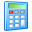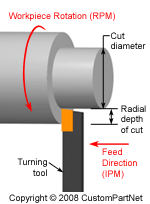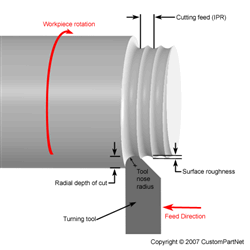Widgets

## Turning Speed and Feed Calculator

 Cutting speed (SFM) × 12 ÷ Cut diameter (in) × π = Spindle speed (RPM) Cutting feed (IPR) × Spindle speed (RPM) = Feed rate (IPM)
 Cut length (in) ÷ Feed rate (IPM) = Cut time (min)## Turning Horsepower Calculator

 Feed rate (IPM) × Radial depth of cut (in) × Cut diameter (in) × π × π = Material removal rate (in³/min)
 Material removal rate (in³/min) × Unit power (hp/in³/min) = Spindle horsepower (hp) Spindle horsepower (hp) ÷ Machine efficiency (%) / 100 = Motor horsepower (hp) Spindle horsepower (hp) × 63,030 ÷ Spindle speed (RPM) = Spindle torque (in∙lb)## Turning Surface Roughness Calculator

 Cutting feed (IPR) ² ÷ Tool nose radius (in) × 24 = Ra surface roughness (μin) ÷ 1000000 Ra surface roughness (μin) × 1.11 = RMS surface roughness (μin)